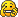This topic will be like the extra math functions thread. Instead of posting math functions you are to post thyme scripts that are useful in certain situations. Here's an example.
Code: Select all
`Scene.my.IsMouseInBox=(P1, P2, CIT, CIF) =>{App.mousePos(0) >= P1(0) && App.mousePos(1) <= P1(1) && App.mousePos(0) <= P2(0) && App.mousePos(1) >= P2(1) ? {CIT} : {CIF}}`

As you can see, using a "mouse over" script isn't very useful for most situations but when the time comes, it can be proven to be very helpful.

TL;DR Post scripts that are useful in certain situations but are usually not needed.

I know that this seems like a somewhat stupid topic, but I think that it could be very helpful. I'll start the thread off with a few of my own. If you have any that you would like to contribute please post them below and I will edit the OP with them. It would also be helpful if you told us what they do and how to use them.

Code: Select all
`Scene.my.IsMouseInBox=(P1, P2, CIT, CIF) =>{App.mousePos(0) >= P1(0) && App.mousePos(1) <= P1(1) && App.mousePos(0) <= P2(0) && App.mousePos(1) >= P2(1) ? {CIT} : {CIF}};//Returns true or false and changes the color of an object if the mouse is within a specified areaScene.my.toBool=(N)=>{N == 0 ? false : true};//Returns true or falsescene.my.toFloat=(N)=>{N + 0.0};//Returns the given number as a floatscene.my.toString=(N)=>{N + ""};//Returns the given value, N, as a stringScene.my.toInt=(N)=>{N - math.mod(N, 1)}'//Returns the given number as an integer//i.e. 10.6(float) scene.my.toInt(10.6) = 10(Integer)//!! Does not return an integer if given a boolean. Using the built in math.toInt in Algodoo will.Scene.my.TimedEvent=(WT, C, What)=>{(sim.time - c) >= WT ? {what} : {}};//Quick way to use a timer. Wt is wait time, C is what part of the object to use as a "dummy", and what is what will happen if true.//For the "what" you need put curly brackets around it like the example below and you must put e.this/other.whateveryouusedforC = sim.time.spawnBoxGrid = (offset, numPerRow, numRows)  => {for(numRows,(y)=>{for(numPerRow,(x)=>{scene.addBox({pos = (offset) + [x,y]})})})};//Spawns a box grid`
Last edited by standardtoaster on Thu Jan 14, 2010 10:51 pm, edited 1 time in total.standardtoaster

Posts: 606
Joined: Mon Aug 31, 2009 7:57 pm

I forgot about my SpawnBoxGrid function that I made last year. I have put it in the OP and this post.
Code: Select all
`spawnBoxGrid = (offset, numPerRow, numRows)  => {for(numRows,(y)=>{for(numPerRow,(x)=>{scene.addBox({pos = (offset) + [x,y]})})})}//Spawns a box grid`standardtoaster

Posts: 606
Joined: Mon Aug 31, 2009 7:57 pmcanadian joe wrote:im just writing this so i can sig it

RicH wrote:Phun's Army.